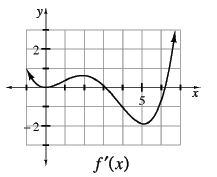### Home > CALC > Chapter Ch9 > Lesson 9.4.2 > Problem9-122

9-122.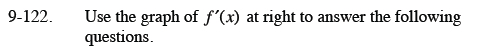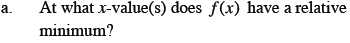This occurs when f′(x) changes from negative to positive.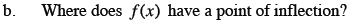This occurs when the slope of f′(x) is 0.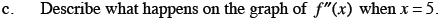What is the slope of the given graph at x = 4.8?
At x = 5?
At x = 5.2?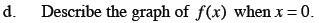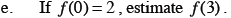$f(3)=f(0)+\int_0^3f^\prime(x)dx$